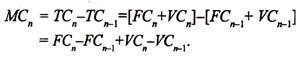# Cost of Production

18/03/2020

### The Determinants of Cost:

The cost of producing any given amount of output by a firm depends on two main factors:

#### (a) The Quantities of Resources and Their Combinations:

The cost to the firm of producing any output evidently depends upon the physical quantities of actual resources or services—labour, material, machine hours, and so forth—used in production. Thus, the cost of producing a tons of steel depends upon the quantities of iron ore, limestone, coal, blast-furnace, etc. used in the production.

As the larger output requires the greater amount of resources, the total cost for larger output becomes large. And the smaller output requires the smaller resources; the total cost for smaller output becomes small. Besides, the total cost for producing a given amount of output becomes small when these resources are combined in optimum proportions.

#### (b) Techniques of Productions:

A firm can produce at low cost when it produces with the new and improved techniques of production. Production with the old and out-dated technique involves higher cost. The profit maximisation requires the use of the particular technique of production which would allow the optimum combination of factors.

In the short period the optimum combination for any given level of output is the least-cost combination possible with the fixed factor units. But this may not be the absolute optimum combination if all the factors could be adjusted. Over the longer period, all factors can be varied, and so the firm is free to select the production technique of factors.

### Three Cost Concepts:

Cost of production refers to the expenses incurred by a business firm in producing a commodity. There are different cost concepts. Three important concepts are total cost, average (total) cost and marginal cost.

#### Total Cost:

It is the total cost of producing a particular output of the commodity in question. It is divided into two parts total fixed cost and total variable cost.

Fixed Costs:

Fixed Costs—also known as ‘overhead cost’ are costs which do not vary with output. These costs will be the same whether output is 1 unit, 10 units, or even 100 units of a commodity. They will be the same even when output is zero. Fixed costs include rent of factory and any office building, insurance charges, interest on bank loans, depreciation of machinery, annual licence fee which is paid to the government and so on.

Variable Costs:

Variable Costs—also known as ‘prime (direct) cost’ are costs which vary with changes in output. The greater the output, the greater will be the variable costs. If output is zero, no variable cost has to be incurred. Variable costs include wages, costs of fuel and power and costs of raw materials.

Total cost is calculated by adding up total fixed cost and total variable cost.

#### Average (Total) Cost:

Average total cost, or simply average cost, refers to the cost per unit of output and is calculated by dividing the total cost by the level of output. Thus if one total cost of producing 10 units of a commodity is Rs. 90, the average cost is Rs. 9 per unit.

Average cost is divided into two parts average fixed cost (i.e., total fixed cost divided by the level of output) and average variable cost (i.e., total variable cost divided by output).

#### Marginal Cost:

It is the addition to total cost incurred by increasing output by one unit. In other words, it is the extra cost of producing on extra unit of output.

MC as change in TVC:

Marginal cost for the nth unit may be expressed asSince fixed cost remains unchanged at all levels of output up to capacity we can write FC = FCn-1 in which case MC may be expressed as:

MCn = VCn – VCn-1

Thus marginal cost refers to marginal variable cost. In other words, MC has no relation to fixed cost.